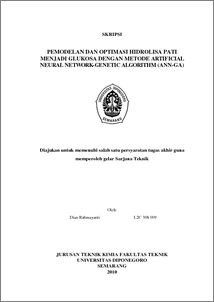# PEMODELAN DAN OPTIMASI HIDROLISA PATI MENJADI GLUKOSA DENGAN METODE ARTIFICIAL NEURAL NETWORK-GENETIC ALGORITHM (ANN-GA)

Rahmayanti, Dian (2010) PEMODELAN DAN OPTIMASI HIDROLISA PATI MENJADI GLUKOSA DENGAN METODE ARTIFICIAL NEURAL NETWORK-GENETIC ALGORITHM (ANN-GA). Undergraduate thesis, Universitas Diponegoro.Preview
PDF - Published Version
1201Kb

## Abstract

Modeling and optimization methods are commonly used, still not able to model and optimize the complex chemical processes non-linear. Hybrid method of Artificial Neural Network-Genetic Algorithm (ANN-GA) is a combination of modeling Artificial Neural Network (ANN) and optimization with Genetic Algorithm (GA) that works simultaneously. The ANN-GA is considered as an effective method for resolving these problems and obtain optimum conditions globally. The aim of this study is to develop a modeling and optimization with ANN-GA hybrid methods, which applied in process of making glucose from starch hydrolysis. The ANN-GA stategy consists of two steps. In the first step, an ANN-based prosess model is developed. Therefore, the input at ANN model will be optimized using GA technique. Variables data that will be used are secondary data research from Baskar et al. (2008). The independent variables are starch concentration, temperature, time and enzyme concentration. The yield of glucose was modeled and optimized as a function of four independent variables using ANN-GA. The optimal values of starch concentration, enzyme concentration, temperature and time with ANN-GA method were 7.1302 % (w/v), 1.4708 %(w/v), 40.5250ºC, and 166.0374 min respectively with predicted glucose yield of 6.0842 mg/mL. These result differed from the secondary data (Baskar et al., 2008) which were used modeling and optimization methods with RSM. This is due to differences in the value of regression coefficient, R2, which is achieved from both methods. The R2 values of ANN-GA method was 0.9755, which means that 97.55% glucose yield variables are represented in the model. While RSM method was only able to achieved value of R2 for 0.842. Since ANN is more accurate and more generalized model than RSM, it is better equipped to reach the global optimum. Modeling and optimization with ANN-GA method can be developed and used to obtain the model in starch hydrolysis into glucose and the optimal operating conditions simultaneouosly.

Item Type: Thesis (Undergraduate) ARTIFICIAL NEURAL NETWORK-GENETIC ALGORITHM T Technology > TP Chemical technology Faculty of Engineering > Department of Chemical EngineeringFaculty of Engineering > Department of Chemical Engineering 18540 Dr. Istadi Istadi 03 Aug 2010 09:34 03 Aug 2010 09:34

Repository Staff Only: item control page